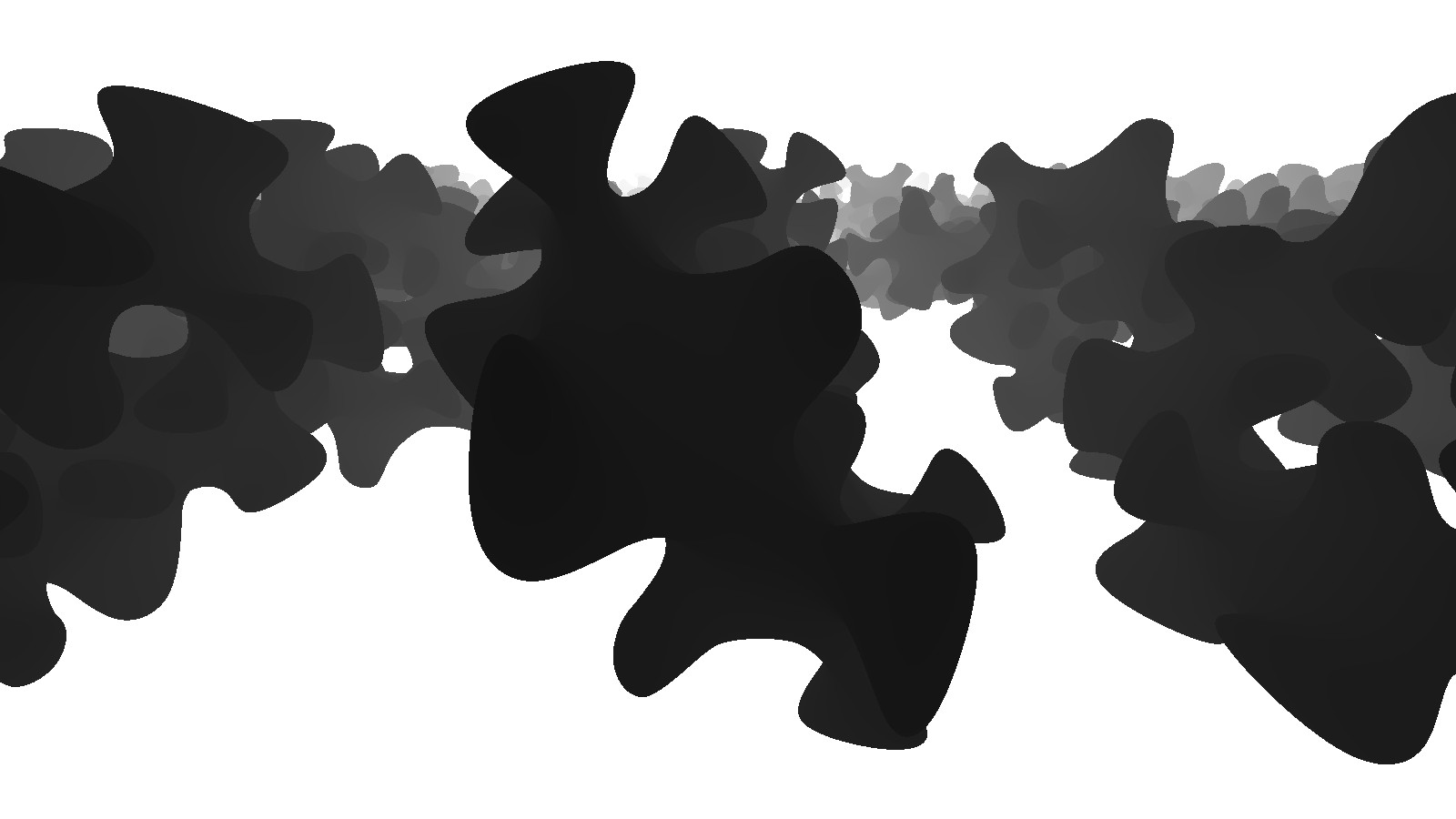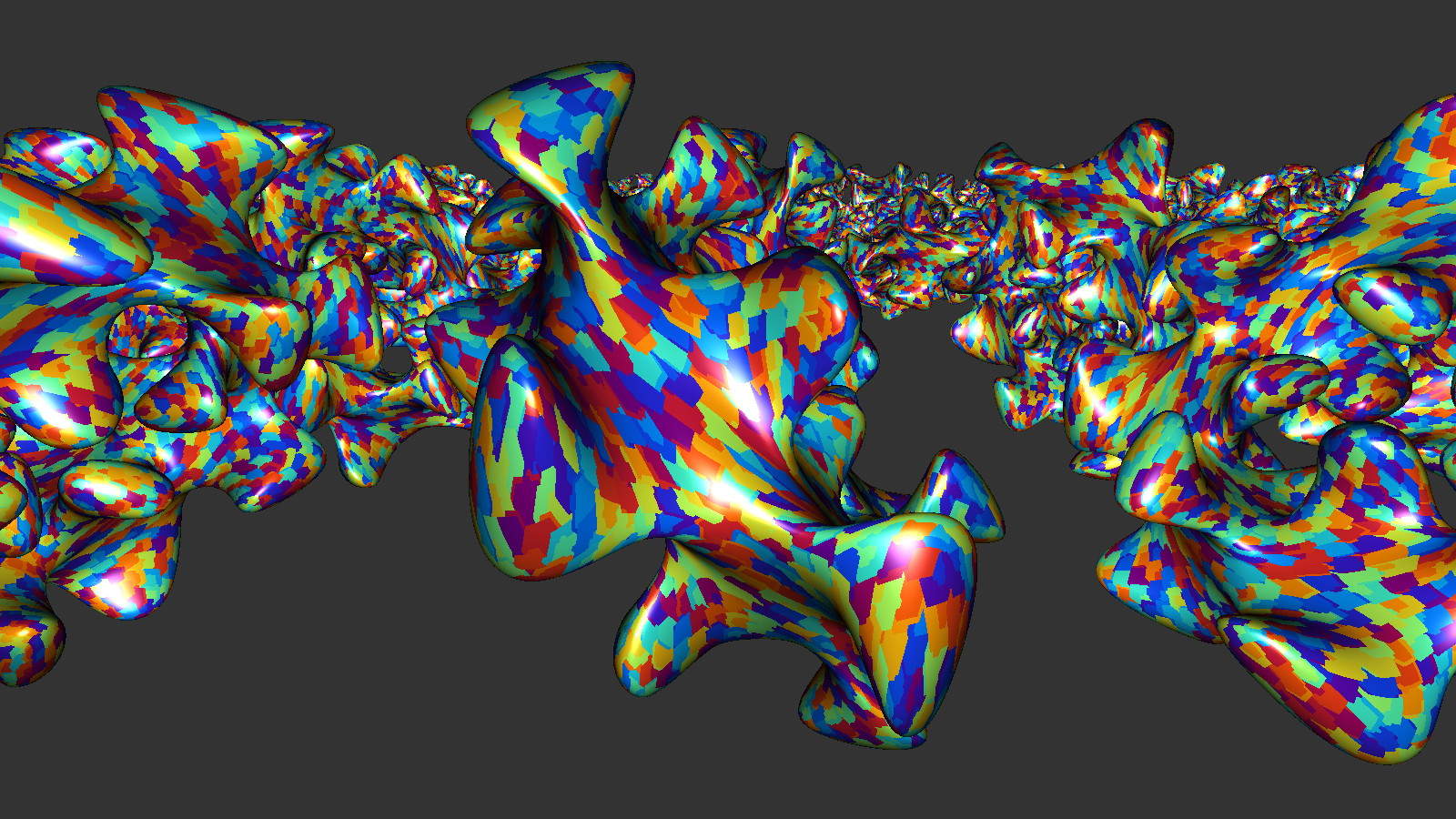September 13, 2021

Compute versus Hardware

It was tempting to compare the Compute Shader rasterization with Mesh Shader and MDI on all supported platforms. Unreal 5 Nanite is already demonstrating outstanding performance with small triangle rendering. But what about numbers from tile-based architectures? And how good is a dedicated rasterizer?

Let’s use our old test Mesh Shader versus MDI with 498990 64×128 Meshlets without any culling except back-face. The data prepared for Meshlet fits well Compute based rasterization. All vertices and indices are independent and tightly packed. It’s possible to spawn a group per Meshlet and rasterize a triangle per thread. We will limit software rasterization to depth-only mode because 64-bit atomics are not available on Mobile devices and Metal. It will not make a big difference in performance. Metal is also not supporting atomic operations with textures, but there is a way to create texture from buffer data and use buffer atomic operations.

We compared single DIP 32-bit indices, Mesh Shader, MultiDrawIndirect, and Compute Shader rasterization performance on Nvidia, AMD, Intel, Qualcomm, and Apple GPUs.

And here are the results:

GeForce 2080 Ti 12.05 B 12.57 B 12.63 B 17.26 B
GeForce 1060 M 3.86 B 3.90 B 4.55 B
Radeon 6700 XT 14.73 B 4.38 B 3.63 B 16.74 B
Radeon RX 5600M 4.87 B 1.11 B 7.57 B
Radeon Vega 56 (macOS) 2.40 B 796.0 M 3.17 B
Apple M1 (macOS) 1.37 B 739.0 M 2.30 B
Apple A14 (iOS) 666.1 M 475.0 M 1.02 B
Intel UHD Graphics 680.0 M 396.5 M 556.1 M
Adreno 660 (Android) 565.2 M 31.17 M 497.3 M

The table demonstrates the number of processed triangles per second (millions and billions).

Well…

• The era of GPU fixed-function units and an enormous number of shader types is almost over.
• MultiDrawIndirect doesn’t work well on mobile because of the tile-based rendering.
• Best Mesh Shaders / MDI are slower than Compute-based rasterization.

What we need are compute shaders and the ability to spawn threads from shaders effectively. Everything else (including raytracing) can be easily implemented on Compute Shader level. Ten years ago, the performance of Intel Larrabee wasn’t enough for compute-only mode, but now it’s possible to discard all other shaders.

A compute shader extension allowing atomically to write payloads into the image will change everything. Without it, we are limited to 32-bit payload data and must perform a redundant triangle intersection.

```uint imageAtomicPayloadMax(gimage2D atomic_image, gimage2D payload_image,
ivec2 P,Here is an unoptimized code of Compute rasterization shader that was used in all tests on all platforms and APIs without any modification thanks to our Clay shader compiler:

```layout(local_size_x = GROUP_SIZE) in;

layout(row_major, binding = 0) uniform common_parameters {
mat4 projection;
mat4 modelview;
vec4 camera;
};
layout(std140, binding = 1) uniform compute_parameters {
uint num_meshlets;
uint group_offset;
vec2 surface_size;
float surface_stride;
};
layout(std140, binding = 2) uniform transform_parameters {
vec4 transforms[NUM_INSTANCES * 3u];
};

layout(std430, binding = 3) readonly buffer vertices_buffer { vec4 vertices_data[]; };
layout(std430, binding = 4) readonly buffer meshlets_buffer { uint meshlets_data[]; };
#if CLAY_MTL
layout(std430, binding = 5) buffer surface_buffer { uint out_surface[]; };
#else
layout(binding = 0, set = 1, r32ui) uniform uimage2D out_surface;
#endif

shared uint num_primitives;
shared uint num_vertices;
shared uint base_index;
shared uint base_vertex;

shared vec4 row_0;
shared vec4 row_1;
shared vec4 row_2;

shared vec3 positions[NUM_VERTICES];
shared uint indices[NUM_PRIMITIVES * 3u];

/*
*/
void rasterize(vec3 p0, vec3 p1, vec3 p2) {

[[branch]] if(p0.z < 0.0f || p1.z < 0.0f || p2.z < 0.0f) return;

vec3 p10 = p1 - p0;
vec3 p20 = p2 - p0;
float det = p20.x * p10.y - p20.y * p10.x;
[[branch]] if(det >= 0.0f) return;

vec2 min_p = floor(min(min(p0.xy, p1.xy), p2.xy));
vec2 max_p = ceil(max(max(p0.xy, p1.xy), p2.xy));
[[branch]] if(max_p.x < 0.0f || max_p.y < 0.0f || min_p.x >= surface_size.x || min_p.y >= surface_size.x) return;

min_p = clamp(min_p, vec2(0.0f), surface_size - 1.0f);
max_p = clamp(max_p, vec2(0.0f), surface_size - 1.0f);

vec2 texcoord_dx = vec2(-p20.y, p10.y) / det;
vec2 texcoord_dy = vec2(p20.x, -p10.x) / det;

vec2 texcoord_x = texcoord_dx * (min_p.x - p0.x);
vec2 texcoord_y = texcoord_dy * (min_p.y - p0.y);

for(float y = min_p.y; y <= max_p.y; y += 1.0f) {
vec2 texcoord = texcoord_x + texcoord_y;
for(float x = min_p.x; x <= max_p.x; x += 1.0f) {
if(texcoord.x >= 0.0f && texcoord.y >= 0.0f && texcoord.x + texcoord.y <= 1.0f) {
float z = p10.z * texcoord.x + p20.z * texcoord.y + p0.z;
#if CLAY_MTL
uint index = uint(surface_stride * y + x);
atomicMax(out_surface[index], floatBitsToUint(z));
#else
imageAtomicMax(out_surface, ivec2(vec2(x, y)), floatBitsToUint(z));
#endif
}
texcoord += texcoord_dx;
}
texcoord_y += texcoord_dy;
}
}

/*
*/
void main() {

uint group_id = gl_WorkGroupID.x + group_offset;
uint local_id = gl_LocalInvocationIndex;

// meshlet parameters
[[branch]] if(local_id == 0u) {
uint transform_index = (group_id / num_meshlets) * 3u;
row_0 = transforms[transform_index + 0u];
row_1 = transforms[transform_index + 1u];
row_2 = transforms[transform_index + 2u];
uint meshlet_index = (group_id % num_meshlets) * 4u;
num_primitives = meshlets_data[meshlet_index + 0u];
num_vertices = meshlets_data[meshlet_index + 1u];
base_index = meshlets_data[meshlet_index + 2u];
base_vertex = meshlets_data[meshlet_index + 3u];
}
memoryBarrierShared(); barrier();

[[unroll]] for(uint i = 0; i < NUM_VERTICES; i += GROUP_SIZE) {
uint index = local_id + i;
[[branch]] if(index < num_vertices) {
uint address = (base_vertex + index) * 2u;
position = vec4(dot(row_0, position), dot(row_1, position), dot(row_2, position), 1.0f);
position = projection * (modelview * position);
positions[index] = vec3(round((position.xy * (0.5f / position.w) + 0.5f) * surface_size * 256.0f) / 256.0f - 0.5f, position.z / position.w);
}
}

[[loop]] for(uint i = local_id; (i << 2u) < num_primitives; i += GROUP_SIZE) {
uint index = i * 12u;
uint address = base_index + i * 3u;
uint indices_0 = meshlets_data[address + 0u];
uint indices_1 = meshlets_data[address + 1u];
uint indices_2 = meshlets_data[address + 2u];
indices[index +  0u] = (indices_0 >>  0u) & 0xffu;
indices[index +  1u] = (indices_0 >>  8u) & 0xffu;
indices[index +  2u] = (indices_0 >> 16u) & 0xffu;
indices[index +  3u] = (indices_0 >> 24u) & 0xffu;
indices[index +  4u] = (indices_1 >>  0u) & 0xffu;
indices[index +  5u] = (indices_1 >>  8u) & 0xffu;
indices[index +  6u] = (indices_1 >> 16u) & 0xffu;
indices[index +  7u] = (indices_1 >> 24u) & 0xffu;
indices[index +  8u] = (indices_2 >>  0u) & 0xffu;
indices[index +  9u] = (indices_2 >>  8u) & 0xffu;
indices[index + 10u] = (indices_2 >> 16u) & 0xffu;
indices[index + 11u] = (indices_2 >> 24u) & 0xffu;
}
memoryBarrierShared(); barrier();

// rasterize triangles
[[branch]] if(local_id < num_primitives) {
uint index = local_id * 3u;
uint index_0 = indices[index + 0u];
uint index_1 = indices[index + 1u];
uint index_2 = indices[index + 2u];
rasterize(positions[index_0], positions[index_1], positions[index_2]);
}
}
```

Reproduction binaries for Windows: TellusimDrawMeshlet.zip